# Color/Tone and Colorcheck Appendix

View legacy documentation

Algorithms and reference formulas

This page contains algorithms and reference formulas for Colorcheck, Color/Tone Setup and Color/Tone Auto. It’s mostly in green text because it’s all math.

### Color difference (error) formulas-

You have two rectangular patches with different colors. How different are they?

The Wikipedia Color Difference page has a concise introduction to color difference concepts and equations. This page (written before the Wikipedia page was available) covers similar material.

The notation on this page is adapted from the Digital Color Imaging Handbook, edited by Gaurav Sharma, published by the CRC Press, referred to below as DCIH. The DCIH online Errata was consulted.

In measuring color error, keep it in mind that accurate color is not necessarily the same as pleasing color. Many manufacturers deliberately alter colors to make them more pleasing, typically by increasing saturation. (That is why Fujichrome Velvia was so successful when it was introduced in 1990.) In calculating color error, you may choose not to use the exact Colorchecker (or other chart) L*a*b* reference values; you may want to substitute your own enhanced values. Imatest Master allows you to enter reference values from a file written in CSV format (you can save the values from a measured chart using Color/Tone Setup).

Imatest users frequently ask about the meaning of “corr” and “uncorr” in the Colorcheck a*b* error plot. Corr means that the mean saturation (mean chroma =  mean(sqrt(a*2+b*2)) of the camera is adjusted to be the same as that of the reference before making the comparison. This is a very easy correction to make; it tells how accurate the color hue would be if the mean chroma were the same as the reference. See below for more detail.

### Absolute differences (including luminance)

#### CIE 1976

The CIELAB (L*a*b*) color space was designed to be relatively perceptually uniform. That means that perceptible color difference is approximately equal to the Euclidean distance between L*a*b* values. For colors {L1*, a1*, b1*} and {L2*, a2*, b2*}, where ΔL* = L2* – L1*, Δa* = a2* – a1*, and Δb* = b2* – b1*,

$$\Delta E^*_{ab} = \sqrt{(\Delta L^*)^2 + (\Delta a^*)^2 + (\Delta b^*)^2 }$$      (DCIH (1.42, 5.35).

Although ΔE*ab is relatively simple to calculate and understand, it’s not very accurate, especially for strongly saturated colors. L*a*b* is not as perceptually uniform as its designers intended. For example, for saturated colors, which have large chroma values (C* = ( a*2 + b*2 )1/2 ), the eye is less sensitive to changes in chroma than to corresponding changes for Hue $$\left( \Delta H^* = \sqrt{(\Delta E^*_{ab})^2 – (\Delta L^*)^2 – (\Delta C^*)^2 } \; \right)$$ or Luminance (ΔL*). To address this issue, several additional color difference formulas have been established. In these formulas, just-noticeable differences (JNDs) are represented by ellipsoids rather than circles.

#### CIE 1994

The CIE-94 color difference formula, ΔE*94, provides a better measure of perceived color difference than ΔE*ab .

ΔE*94 = (L*)2 + (ΔC*SC )2 + (ΔH* ⁄ SH )2 )1/2 (DCIH (5.37); omitting constants set to 1 ), where

SC = 1 + 0.045 C* ; SH = 1 + 0.015 C*   (DCIH (1.53, 1.54) )

[ C* = ( ( a1*2 + b1*2 )1/2 ( a2*2 + b2*2 )1/2 )1/2 (the geometrical mean chroma) gives symmetrical results for colors 1 and 2. However, when one of the colors (denoted by subscript s) is the standard, the chroma of the standard, Cs* = ( as*2 + bs*2 )1/2, is preferred for calculating SC and SH. The asymmetrical equation is used by Bruce Lindbloom.]

ΔH* = (E*ab)2 – (ΔL*)2 – (ΔC*)2 )1/2    (hue difference ; DCIH (5.36) )

ΔC* = ( a1*2 + b1*2 )1/2 – ( a2*2 + b2*2 )1/2   (chroma difference)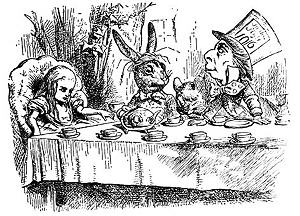An early meeting of the CMC Standards Committee

#### CMC

The CMC color difference formula is used by the textile industry to match bolts of cloth, but it hasn’t gained traction in the photographic industry. It is slightly asymmetrical: subscript s denotes the standard (reference) measurement. CMC is the Color Measurement Committee of the Society of Dyers and Colourists (UK). We keep it in Imatest only for backwards compatibility

ΔE*CMC(l,c) = ( (ΔL* ⁄ lSL)2 + (ΔC*cSC )2 + (ΔH* ⁄ SH )2 )1/2 (DCIH (5.37) ), where

(That’s the lowercase letter l in (l,c) and the denominator of (ΔL* ⁄ lSL)2.) ΔE*CMC(1,1) (l = c = 1) is used for graphic arts perceptibility mesurements. l = 2 is used in the textile industry for acceptibility of fabric matching. For now Imatest displays ΔE*CMC(1,1).

SL = 0.040975 Ls* ⁄ (1+0.01765 Ls*) ; Ls* ≥ 16 (DCIH (1.48) ) = 0.511 ; Ls* < 16

SC = 0.0638 Cs* ⁄ (1+0.0131 ) + 0.638 ; SH = SC (TCMC FCMC + 1 – FCMC ) (DCIH (1.49, 1.50) )

FCMC = ( ( Cs*)4 ⁄ ( ( Cs*)4 + 1900 ) )1/2 (DCIH (1.51);

TCMC = 0.56 + | 0.2 cos(hs* + 168°) | 164° ≤ hs* ≤ 345° (DCIH (1.52) ) = 0.36 + | 0.4 cos(hs* + 35 °) | otherwise

ΔH* and ΔC* have the same formulas as CIE-94.

#### CIEDE2000

The CIEDE2000 formulas (ΔEoo and ΔCoo ) are the best current color difference metric, and may be regarded as more accurate than the previous formulas. We omit the equations here because they are given in the Wikipedia Color Difference page and in Gaurav Sharma’s CIEDE2000 Color-Difference Formula web page. Default values of 1 are used for parameters kL, kC, and kH.

The CIE 1976 color difference metrics (ΔE*ab…) are still the most familiar. CIE 1994 is more accurate and robust, and retains a relatively simple equation. The complexity of the CIEDE2000 equations (DCIH, section 1.7.4, pp. 34-40) has slowed its widespread adoption, but it’s now the accepted standard. CIEDE2000 color difference metrics are the best choice.

### Chroma differences ΔC (color differences that omit luminance difference)

Exposure errors strongly affect color differences ΔE*ab, ΔE*94, and ΔE*00. Since it is useful to look at color errors independently of exposure error, we define color differences that omit ΔL*.

$$\Delta C_{ab} = \sqrt{(\Delta a^*)^2 + (\Delta b^*)^2} = \sqrt{(\Delta E^*_{ab})^2 – (\Delta L^*)^2 }$$     (This is a more general form: Delta-E with Delta-L removed.)

$$\Delta C_{94} = \sqrt{(\Delta C^* / S_C)^2 + (\Delta H^* / S_H)^2}$$

ΔC00 omits the ( ΔL’ ⁄ kLSL )2 term from the ΔE00 (square root) equation (see Sharma).

These formulas don’t entirely remove the effects of exposure error since a* and b* are affected somewhat by exposure, but they reduce it to a manageable level. Note that the ΔC definition can cause some confusion because it is different from the pure chroma difference, Δ|C*|, where chroma = |C*| = sqrt(a*2 + b*2). Δ|C*| is not suitable as a perceptual measurement because it does not include hue.

### Color difference representation (ellipses)

Color difference can be represented in Imatest by ΔC circles or ellipses on the a*b* plane of L*a*b* space. It can also be displayed in the xy and uv representations— useful for visualizing the perceptual uniformity of these spaces (uv isn’t as good as claimed), but a*b* is usually the best representation. Three ΔC representations are shown below (click on images to see them full size). ΔC94 and ΔC2000 are close to each other, but very different from (and superior to) ΔCab.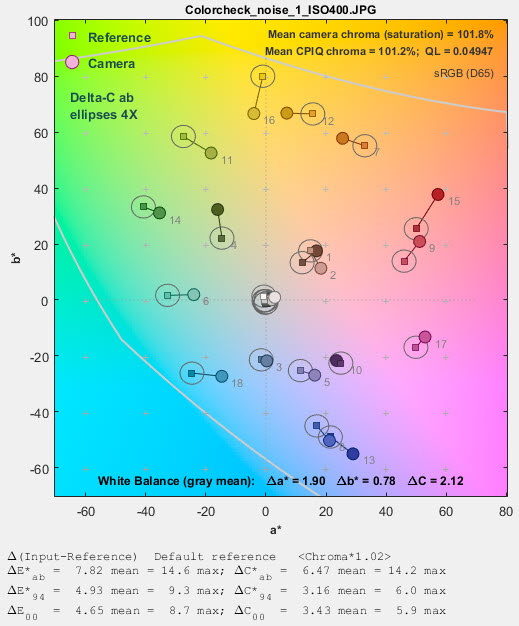ΔCab circlesΔC94 ellipses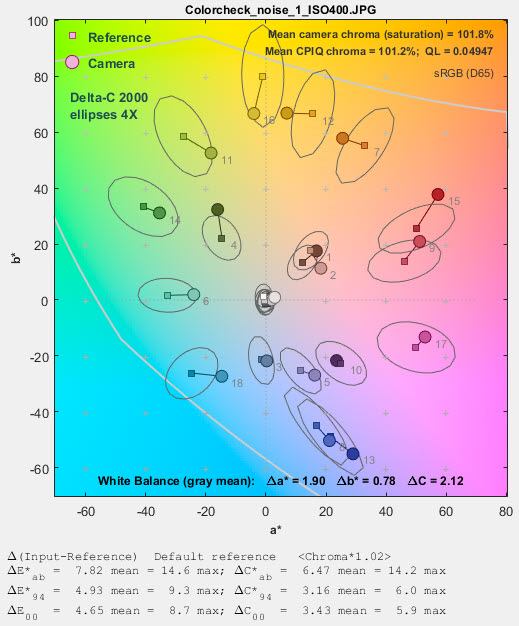ΔC2000 ellipses

Until these ellipses were added to Imatest they were not generally available. We thought the difference between ΔC94 and ΔC2000 was greater than it actually is. In Color/Tone Setup these ellipses can be set in the Color ellipses dropdown menu. Color/Tone Auto uses settings saved from Color/Tone Setup. In Colorcheck they can be selected in the Settings window.

### Color difference visualizer

An app for visualizing color differences has been added to Imatest 5.2. It works best viewed on a high quality calibrated monitor with at least sRGB gamut, though it can provide information on colors on lower quality monitors. To open it from the Imatest main window, press Utility, Color Diff Visualizer or press Color Diff Visualizer in the Help tab in the box on the right. This opens the window below with a* = b* = 0 in the large display on the left and Δa* = Δb* = 0 in the small display at the bottom, which illustrates the rotation function.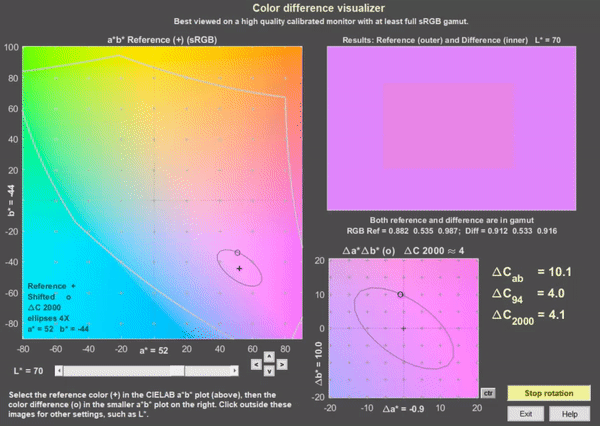Color difference visualizer, shown for a* = 52, b* = 44, rotating on ΔC2000 ≅ 4 ellipse

To view a color difference, select an a*,b* reference value by clicking on the large a*b* display on the left. (a* and b* will be rounded to the nearest integer.) Then select Δa* and Δb* by clicking on the small display on the bottom. Color difference results are displayed on the top-right. In both the a*b* and Δa*Δb* displays + indicates the a*, b* reference value and o indicates Δa*, Δb* difference values. The selected values are displayed near the x and y-axes, respectively.

In the results display, the outer region is the a*, b* reference value (shown as magenta) and the inner part is the a*+Δa*, b*+Δb* shifted value (shown above rotating on the ΔC2000 ≅ 4 ellipse).

The small buttons with ^, v, <, and > below the right of the a*b* display shift the a* or b* setting by 1. The small ctr button to the right of the Δa*Δb* display sets Δa* = Δb* = 0.

sRGB color space is assumed. The middle gray curves in the large a*b* image represent the gamut boundaries for sRGB with L (HSL) = 0.5. Note that this is not the same as CIELAB L* = 50 (it’s more difficult to find a curve for this value).

The L* slider lets you set luminance L*.

The Rotate on ellipse button on the lower-right causes the Δa* and Δb* values to move around the ellipse. The default ellipse is for ΔC2000 = 4.  You can pause or resume rotation by pressing the button. You can check (or uncheck) Fast rotation in the Settings dropdown menu. Color difference visibility may be larger during rotation than for static images because of the way the eye adapts.

The Settings dropdown lets you reset default values ( a* = Δa* = b* = Δb* =0 and L* = 70), set the axes limits in the Δa*Δb* window to ±20 (the default) or ±10, or select Fast rotation.

The Color ellipses dropdown let you set the ellipse type (none, Macadam, ΔCab, ΔC94, ΔC00 (ΔC2000) and magnification (typically 4x or 2x for the ΔC ellipses; 10x or 5x for the Macadam ellipses; 1x is just too small to be useful). ΔC2000 is the best available color difference formula, though it’s far from perfect.

### Color differences corrected for chroma (saturation) boost/cut

Many digital cameras deliberately boost chroma, i.e., saturation, to enhance image appearance in digital cameras. This boost increases color error in the ΔE* and ΔC formulas, above, though it may improve perceived image quality if it’s not overdone.

The mean chroma percentage is

Chroma(%) = 100% * (measured mean( (ai*2+bi*2)1/2 ) ) ⁄ (Colorchecker mean( (ai*2+bi*2)1/2 ) )

= 100% * mean (|Cmeasured|) ⁄ mean (|Cideal|) ; |Ci| = (ai*2+bi*2 )1/2

1 ≤ i ≤ 18 (the first three rows of the Colorchecker)

Chroma, which is closely related to the perception of saturation, is boosted when Chroma(%) > 100. Chroma boost increases ΔE* and ΔC* color error measurements. Since it is easy to remove chroma boost in image editors (with saturation settings), it is useful to measure the color error after the mean chroma has been corrected (normalized) to 100%. To do so, normalized ai_corr and bi_corr are substituted for measured (camera) values ai and bi in the above equations.

ai_corr = 100 aiChroma(%) ; bi_corr = 100 biChroma(%)

The reference values for the ColorChecker are unchanged. Color differences corrected for chroma are denoted ΔC*ab(corr), ΔC*94(corr), and ΔC*2000(corr).

### Mean and RMS values

Colorcheck Figure 3 reports the mean and RMS values of ΔC*ab corrected (for saturation) and uncorrected, where

mean(x) = Σxin for n values of x.

RMS(x) = σ(x) = (Σxi2n )1/2 for n values of x.

The RMS value is of interest because it gives more weight to the larger errors.

### Colorcheck Algorithm

• Locate the regions of interest (ROIs) for the 24 ColorChecker zones.
• Calculate statistics for the six grayscale patches in the bottom row, including the average pixel levels and a second order polynomial fit to the pixel levels in the ROIs this fit is subtracted from the pixel levels for calculating noise. It removes the effects of nonuniform illumination. Calculate the noise for each patch.
• Using the average pixel values of grayscale zones 2-5 in the bottom (omitting the extremes: white and black), the average pixel response is fit to a mathematical function (actually, two functions). This requires some explanation.

A simplified equation for a capture device (camera or scanner) response is,

normalized pixel level = (pixel level255) = k1 exposuregamc

Gamc is the gamma of the capture device. Monitors also have gamma = gamm defined by

monitor luminance = (pixel level255)gamm

Both gammas affect the final image contrast,

System gamma = gamc * gamm

Gamc is typically around 0.5 = 1/2 for digital cameras. Gamm is 1.8 for Macintosh systems; gamm is 2.2 for Windows systems and several well known color spaces (sRGB, Adobe RGB 1998, etc.). Images tend to look best when system gamma is somewhat larger than 1.0, though this doesn’t always hold certainly not for contrasty scenes. For more on gamma, see Glossary, Using Imatest SFR, and Monitor calibration.

Using the equation, density = – log10(exposure) + k,

log10(normalized pixel level) = log10( k1 exposuregamc ) = k2 – gamc * density

This is a nice first order equation with slope gamc, represented by the blue dashed curves in the figure. But it’s not very accurate. A second order equation works much better:

log10(normalized pixel level) = k3 + k4 * density + k5 * density2

k3, k4, and k5 are found using second order regression and plotted in the green dashed curves. The second order fit works extremely well.

• Saturation S in HSV color representation is defined as S(HSV) = (max(R,G,B)-min(R,G,B))max(R,G,B). S correlates more closely with perceptual White Balance error in HSV representation than it does in HSL.

The the equation for saturation boost in the lower image of the third figure is S’ = (1-e-4S )(1-e-4), where e = 2.71828…

### Grayscale levels and exposure error

Colorchecker grayscale patch densities (in the bottom row) are specified as 0.05, 0.23, 0.44, 0.70, 1.05, and 1.50. Using the equation, pixel level = 255 * (10–density ⁄ 1.06)(1/2.2) for gamma = 2.2 color spaces (see ISO sensitivity, below), the ideal pixel levels would be 236, 195, 157, 119, 83, and 52, about 3% lower than the values measured by Bruce Lindbloom (242, 201, 161, 122, 83, and 49 for the Green channel) and provided with a Colorchecker purchased in October 2005 (243, 200, 160, 122, 85, 52). On the average, these measured values fit the equation,

pixel level = 255 * (10–density ⁄ 1.01)gamma  where gamma = 1/2.2 for common color spaces (sRGB, etc.)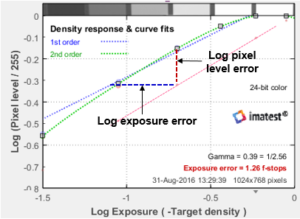Colorchecker Exposure error is measured by comparing the measured pixel levels of patches 2-5 in the bottom row (20-23 in the chart as a whole) with the selected reference levels. White and black patches 1 and 6 of the bottom row (19 and 24) are omitted because they frequently clip.

Gamma is the average slope of log pixel level vs. Log exposure, measured from patches 2-5 in the bottom row, shown on the right as a diagonal dotted blue line (·········). Pixel level is approximately proportional to exposuregamma, hence log10(exposure) is proportional to log10(pixel level) ⁄ gamma, the log exposure error for an individual patch is

Δ(log exposure) = (log10(measured pixel level) – log10(reference level)) ⁄ gamma

Using the mean value of Δ(log exposure) for patches 2-5 and the equation, f-stops = 3.32 * log exposure,

Exposure error in f-stops = 3 32 * (mean(log10(measured pixel level) – log10(reference level))) ⁄ gamma

For Stepchart linear grayscale charts, patches 4 & larger are used for calculating Exposure error.

 Changes in Imatest 5.1+ after December 2018 Prior to December 2018 the specified color space encoding gamma (typically near 1/2.2) was used for gamma (above). The Exposure error estimates for images encoded with gamma close to specified value were quite good, but decoded raw images with gamma ≅ 1 were seriously in error. After December 2018 the Exposure error calculation uses measured values of gamma (rather than the standard value for the color space). Exposure error is now much more consistent (though it will be somewhat different from the old values.)

### ISO sensitivity

Two types of ISO sensitivity are calculated: Saturation-based Sensitivity and Standard Output Sensitivity. These measurements are described on the ISO Sensitivity page.

### Results (CSV and JSON)

Not all results, for example, ΔH, are displayed well graphically, but they can be found in the CSV and JSON text file output. Here is a sample for the Rezchecker chart (similar colors to the Colorchecker), which can be run in Color/Tone.

JSON

“Delta_E”: [9.51,3.87,7.3,6.78,25.1,7.46,12.9,12.6,14,12.5,11.3,11.3,15.4,16.2,18.6,11.1,7.85,5.84,2.93,17.2,12.8,7.67,12.8,10.2,8.13,15.3,11.7,12.7,7.27,15.3],
“Delta_C”: [6.14,1.49,5.76,5.08,22,2.11,6.5,9.51,9.91,9.34,8.15,6.63,4.87,12.2,14.5,5.7,6.36,5.46,2.8,8.94,9.78,4.44,5.59,3.04,2.75,7.83,10.4,10.7,7.11,7.86],
“Delta_E94”: [7.97,3.64,6.06,5.22,17.2,7.22,11.4,9.42,10.3,12.2,11.2,11.2,15.4,11,12.5,11,7.65,5.8,2.91,15.6,9.7,7.59,12.7,10.1,8.11,13.4,7.91,8.18,2.74,13.7],
“Delta_C94”: [3.27,0.696,4.07,2.67,12,0.971,1.96,4.49,2.66,8.87,7.94,6.43,4.67,3,4.15,5.47,6.11,5.42,2.78,5.21,5.08,4.3,5.41,2.96,2.71,2.38,5.84,4.4,2.3,3.94],
“Delta_E2000”: [7.06,3.07,7.22,4.88,16.9,7.14,11.1,8.23,7.68,9.22,8.56,8.47,11.6,10.1,11.1,9.26,6.91,5.29,2.79,13.8,9.44,6.54,9.48,6.89,5.62,12.8,8.68,7.31,2.52,10.6],
“Delta_C2000”: [3.7,0.697,5.72,2.71,12.4,1.06,2.04,4.82,3.12,7.69,6.94,5.85,4.49,3.24,4.6,5.24,5.6,4.86,2.69,7.25,4.61,4.27,4.88,2.87,3.13,2.52,7.72,5.37,2.26,4.45],
“Delta_L”: [57.1,-7.26,-3.57,-4.48,-4.49,61.2,-12.2,48.5,84.2,85.3,79.2,-7.15,-11.2,45.9,44.8,47.1,80.4,-8.28,-9.9,-8.35,-7.85,-9.11,-14.6,-10.6,-11.8,-9.55,-4.6,2.08,-0.832,-14.7,-8.26,6.26,11.5,9.7,7.65,-13.1,51.9,-5.34,6.9,1.49,-13.2,45.8],
“Delta_Chroma”: [5.59,-6.07,1.48,-2.44,-4.45,5.11,-12.8,7.87,7.94,8.31,6.34,-2.11,-6.47,7.72,7.51,6.9,6.43,-8.38,-9.05,9.18,7.7,6,4.07,-12.1,-14.1,4.64,5.46,5.31,2.56,-0.637,-4.9,4.08,5.59,2.41,1.67,-7.77,8.4,1.63,6.55,6.49,-7.04,3.91],
“Delta_Hue_Distance”: [0,0.967,0.213,5.22,2.45,0,17.8,0,0,0,0,0.165,0.594,0,0,0,0,4.48,4.04,0,0,0,0,0.958,3.13,0,0,0,0,8.92,8.46,0,0,0,0,0.899,0,10.3,8.48,2.91,3.51,0],
“Delta_Hue_Degrees”: [0,-3.31,0.456,14,5.76,0,31.3,0,0,0,0,0.374,0.694,0,0,0,0,-8.88,3.02,0,0,0,0,-0.88,3.42,0,0,0,0,10.8,-10.3,0,0,0,0,-1.07,0,11.4,6.56,-2.6,-8.1,0],
“mean_Delta_E”: [11.5],
“mean_Delta_C”: [7.43],
“mean_Delta_E94”: [9.63],
“mean_Delta_C94”: [4.41],
“mean_Delta_E2000”: [8.34],
“mean_Delta_C2000”: [4.56],
“mean_Delta_L”: [13.7],
“mean_Delta_Chroma”: [1.39],
“mean_Delta_Hue_Distance”: [1.99],
“mean_Delta_Hue_Degrees”: [2.93],
“max_Delta_E”: [25.1],
“max_Delta_C”: ,
“max_Delta_E94”: [17.2],
“max_Delta_C94”: ,
“max_Delta_E2000”: [16.9],
“max_Delta_C2000”: [12.4],
“max_Delta_L”: [85.3],
“max_Delta_Chroma”: [9.18],
“max_Delta_Hue_Distance”: [17.8],
“max_Delta_Hue_Degrees”: [31.3],

#### CSV

 Color errors for reference vs input patches Patch Delta-E* Delta-C Delta-E*94 Delta-C 94 Delta-E*CMC Delta-C CMC Delta-E 00 Delta-C 00 Row 2 9.51 6.14 7.97 3.27 8.61 3.94 7.06 3.7 1 3 3.87 1.49 3.64 0.7 2.99 0.83 3.07 0.7 1 4 7.3 5.76 6.06 4.07 6.37 4.85 7.22 5.72 1 5 6.78 5.08 5.22 2.67 5.3 2.94 4.88 2.71 1 7 25.13 21.96 17.16 12.04 16.37 12.01 16.92 12.37 2 12 7.46 2.11 7.22 0.97 6.32 1.13 7.14 1.06 2 13 12.94 6.5 11.35 1.96 10.29 2.49 11.13 2.04 3 18 12.61 9.51 9.42 4.49 8.04 4.84 8.23 4.82 3 19 14.01 9.91 10.26 2.66 8.06 3.64 7.68 3.12 4 20 12.52 9.34 12.18 8.87 14.28 13.09 9.22 7.69 4 21 11.32 8.15 11.17 7.94 13.2 12.02 8.56 6.94 4 22 11.27 6.63 11.15 6.43 11.62 9.65 8.47 5.85 4 23 15.43 4.87 15.37 4.67 12.68 6.87 11.62 4.49 4 24 16.17 12.19 11.04 3 9.63 4.21 10.12 3.24 4 25 18.63 14.45 12.47 4.15 12.83 5.5 11.07 4.6 5 26 11.12 5.7 11.01 5.47 10.87 8.01 9.26 5.24 5 27 7.85 6.36 7.65 6.11 9.78 9.01 6.91 5.6 5 28 5.84 5.46 5.8 5.42 8.64 8.42 5.29 4.86 5 29 2.93 2.8 2.91 2.78 4.41 4.32 2.79 2.69 5 30 17.17 8.94 15.55 5.21 16.51 6.26 13.76 7.25 5 31 12.8 9.78 9.7 5.08 8.65 4.76 9.44 4.61 6 32 7.67 4.44 7.59 4.3 10.26 6.45 6.54 4.27 6 33 12.79 5.59 12.71 5.41 23.74 8.17 9.48 4.88 6 34 10.17 3.04 10.14 2.96 19.49 4.41 6.89 2.87 6 35 8.13 2.75 8.11 2.71 15.51 4.09 5.62 3.13 6 36 15.29 7.83 13.35 2.38 12.18 3.04 12.82 2.52 6 38 11.7 10.42 7.91 5.84 9.71 6.99 8.68 7.72 7 39 12.74 10.71 8.18 4.4 8.02 6.04 7.31 5.37 7 40 7.27 7.11 2.74 2.3 2.98 2.75 2.52 2.26 7 41 15.34 7.86 13.75 3.94 16.52 4.45 10.58 4.45 7 Delta-E* Delta-C Delta-E*94 Delta-C 94 Delta-E*CMC Delta-C CMC Delta-E 00 Delta-C 00 Mean 11.46 7.43 9.63 4.41 10.79 5.84 8.34 4.56 Maximum 25.13 21.96 17.16 12.04 23.74 13.09 16.92 12.37 Additional color errors and values Patch Delta-L* Delta-Chroma |Delta-Hue dist| |Delta-Hue degs| Note: Delta-Hue is undefined for grayscale patches (a* & b* near 0) 2 57.12 5.59 0 0 3 -7.26 -6.07 0.97 -3.31 4 -3.57 1.48 0.21 0.46 5 -4.48 -2.44 5.22 13.97 7 -4.49 -4.45 2.45 5.76 12 61.23 5.11 0 0 13 -12.22 -12.79 17.85 31.31 18 48.52 7.87 0 0 19 84.23 7.94 0 0 20 85.25 8.31 0 0 21 79.24 6.34 0 0 22 -7.15 -2.11 0.17 0.37 23 -11.18 -6.47 0.59 0.69 24 45.95 7.72 0 0 25 44.78 7.51 0 0 26 47.11 6.9 0 0 27 80.38 6.43 0 0 28 -8.28 -8.38 4.48 -8.88 29 -9.9 -9.05 4.04 3.02 30 -8.35 9.18 0 0 31 -7.85 7.7 0 0 32 -9.11 6 0 0 33 -14.64 4.07 0 0 34 -10.62 -12.15 0.96 -0.88 35 -11.75 -14.11 3.13 3.42 36 -9.55 4.64 0 0 38 -4.6 5.46 0 0 39 2.08 5.31 0 0 40 -0.83 2.56 0 0 41 -14.66 -0.64 8.92 10.84 Delta-L* Delta-Chroma |Delta-Hue dist| |Delta-Hue degs| Mean 13.73 1.39 1.99 2.93 Maximum 85.25 9.18 17.85 31.31 Mean camera chroma % 107.1 Mean chroma level CPIQ % 89.96